# Fast Marching in 2D¶

Important: Please read the installation page for details about how to install the toolboxes. $\newcommand{\dotp}{\langle #1, #2 \rangle}$ $\newcommand{\enscond}{\lbrace #1, #2 \rbrace}$ $\newcommand{\pd}{ \frac{ \partial #1}{\partial #2} }$ $\newcommand{\umin}{\underset{#1}{\min}\;}$ $\newcommand{\umax}{\underset{#1}{\max}\;}$ $\newcommand{\umin}{\underset{#1}{\min}\;}$ $\newcommand{\uargmin}{\underset{#1}{argmin}\;}$ $\newcommand{\norm}{\|#1\|}$ $\newcommand{\abs}{\left|#1\right|}$ $\newcommand{\choice}{ \left\{ \begin{array}{l} #1 \end{array} \right. }$ $\newcommand{\pa}{\left(#1\right)}$ $\newcommand{\diag}{{diag}\left( #1 \right)}$ $\newcommand{\qandq}{\quad\text{and}\quad}$ $\newcommand{\qwhereq}{\quad\text{where}\quad}$ $\newcommand{\qifq}{ \quad \text{if} \quad }$ $\newcommand{\qarrq}{ \quad \Longrightarrow \quad }$ $\newcommand{\ZZ}{\mathbb{Z}}$ $\newcommand{\CC}{\mathbb{C}}$ $\newcommand{\RR}{\mathbb{R}}$ $\newcommand{\EE}{\mathbb{E}}$ $\newcommand{\Zz}{\mathcal{Z}}$ $\newcommand{\Ww}{\mathcal{W}}$ $\newcommand{\Vv}{\mathcal{V}}$ $\newcommand{\Nn}{\mathcal{N}}$ $\newcommand{\NN}{\mathcal{N}}$ $\newcommand{\Hh}{\mathcal{H}}$ $\newcommand{\Bb}{\mathcal{B}}$ $\newcommand{\Ee}{\mathcal{E}}$ $\newcommand{\Cc}{\mathcal{C}}$ $\newcommand{\Gg}{\mathcal{G}}$ $\newcommand{\Ss}{\mathcal{S}}$ $\newcommand{\Pp}{\mathcal{P}}$ $\newcommand{\Ff}{\mathcal{F}}$ $\newcommand{\Xx}{\mathcal{X}}$ $\newcommand{\Mm}{\mathcal{M}}$ $\newcommand{\Ii}{\mathcal{I}}$ $\newcommand{\Dd}{\mathcal{D}}$ $\newcommand{\Ll}{\mathcal{L}}$ $\newcommand{\Tt}{\mathcal{T}}$ $\newcommand{\si}{\sigma}$ $\newcommand{\al}{\alpha}$ $\newcommand{\la}{\lambda}$ $\newcommand{\ga}{\gamma}$ $\newcommand{\Ga}{\Gamma}$ $\newcommand{\La}{\Lambda}$ $\newcommand{\si}{\sigma}$ $\newcommand{\Si}{\Sigma}$ $\newcommand{\be}{\beta}$ $\newcommand{\de}{\delta}$ $\newcommand{\De}{\Delta}$ $\newcommand{\phi}{\varphi}$ $\newcommand{\th}{\theta}$ $\newcommand{\om}{\omega}$ $\newcommand{\Om}{\Omega}$

This tour explores the use of Fast Marching methods in 2-D.

In :
warning off
warning on


## Shortest Path for Isotropic Metrics¶

Shortest paths are 2D curves that minimize a weighted length according to a given metric $W(x)$ for $x \in [0,1]^2$. The metric is usually computed from an input image $f(x)$.

The length of a curve $t \in [0,1] \mapsto \gamma(t) \in [0,1]^2$ is $$L(\gamma) = \int_0^1 W(\gamma(t)) \norm{\gamma'(t)} \text{d} t.$$

Note that $L(\gamma)$ is invariant under re-parameterization of the curve $\gamma$.

A geodesic curve $\gamma$ between two points $x_0$ and $x_1$ has minimum length among curves joining $x_0$ and $x_1$, $$\umin{\ga(0)=x_0, \ga(1)=x_1} L(\ga).$$ A shortest curve thus tends to pass in areas where $W$ is small.

The geodesic distance between the two points is then $d(x_0,x_1)=L(\gamma)$ is the geodesic distance according to the metric $W$.

## Pixel values-based Geodesic Metric¶

The geodesic distance map $D(x)=d(x_0,x)$ to a fixed starting point $x_0$ is the unique viscosity solution of the Eikonal equation $$\norm{ \nabla D(x)} = W(x) \qandq D(x_0)=0.$$

This equation can be solved numerically in $O(N \log(N))$ operation on a discrete grid of $N$ points.

We load the input image $f$.

In :
clear options;
n = 300;
f = rescale( load_image(name, n) );


Display the image.

In :
clf;
imageplot(f);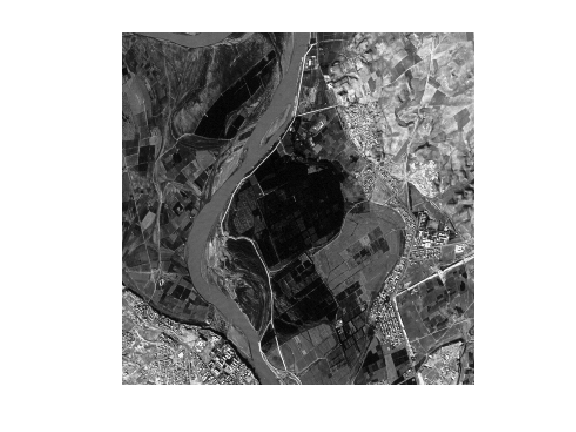Define start and end points $x_0$ and $x_1$ (note that you can use your own points).

In :
x0 = [14;161];
x1 = [293;148];


The metric is defined according to $f$ in order to be low at pixel whose value is close to $f(x)$. A typical example is $$W(x) = \epsilon + \abs{f(x_0)-f(x)}$$ where the value of $\epsilon>0$ should be increased in order to obtain smoother paths.

In :
epsilon = 1e-2;
W = epsilon + abs(f-f(x0(1),x0(2)));


Display the metric $W$.

In :
clf;
imageplot(W);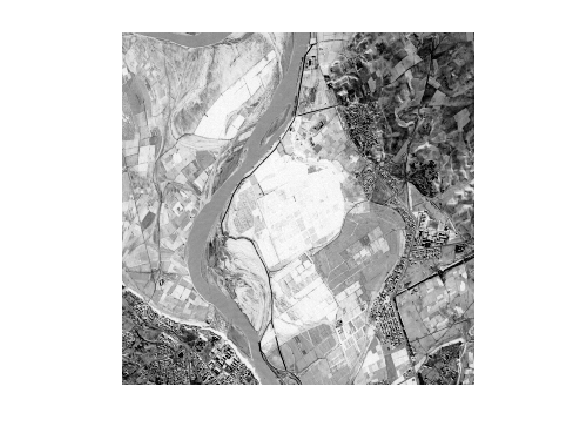Set options for the propagation: infinite number of iterations, and stop when the front hits the end point.

In :
options.nb_iter_max = Inf;
options.end_points = x1;


Perform the propagation, so that $D(a,b)$ is the geodesic distance between the pixel $x_1=(a,b)$ and the starting point $x_0$. Note that the function |perform_fast_marching| takes as input the inverse of the metric $1/W(x)$.

In :
[D,S] = perform_fast_marching(1./W, x0, options);


Display the propagated distance map $D$. We display in color the distance map in areas where the front has propagated, and leave in black and white the area where the front did not propagate.

In :
clf;
hold on;
imageplot( convert_distance_color(D,f) );
h = plot(x0(2),x0(1), '.r'); set(h, 'MarkerSize', 25);
h = plot(x1(2),x1(1), '.b'); set(h, 'MarkerSize', 25);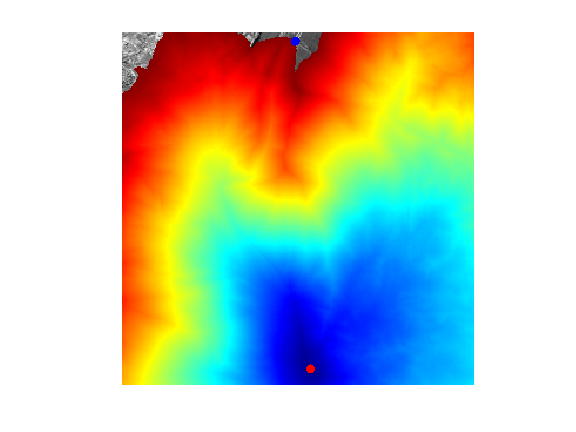Exercise 1

Using |options.nb_iter_max|, display the progressive propagation. This corresponds to displaying the front $\enscond{x}{D(x) \leq t}$ for various arrival times $t$.

In :
exo1()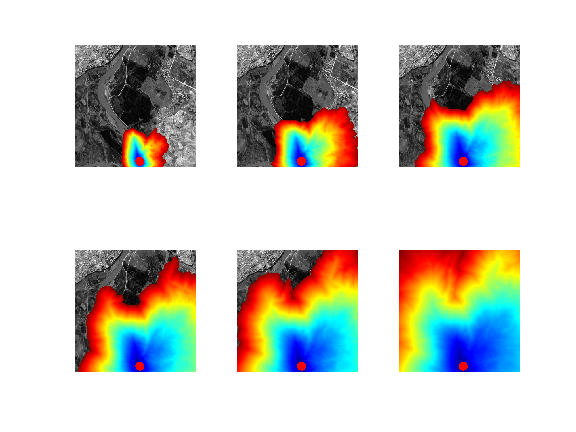In :
%% Insert your code here.


## Geodesic Curve Extraction¶

Once the geodesic distance map $D(x)$ to a starting point $x_0$ is computed, the geodesic curve between any point $x_1$ and $x_0$ extracted through gradient descent $$\ga'(t) = - \eta_t \nabla D(\ga(t)),$$ where $\eta_t>0$ controls the parameterization speed of the resulting curve. To obtain unit speed parameterization, one can use $\eta_t = \norm{\nabla D(\ga(t))}^{-1}$.

Recompute the geodesic distance map $D$ on the whole grid.

In :
options.nb_iter_max = Inf;
options.end_points = [];
[D,S] = perform_fast_marching(1./W, x0, options);


Display $D$.

In :
clf;
imageplot(D);
colormap jet(256);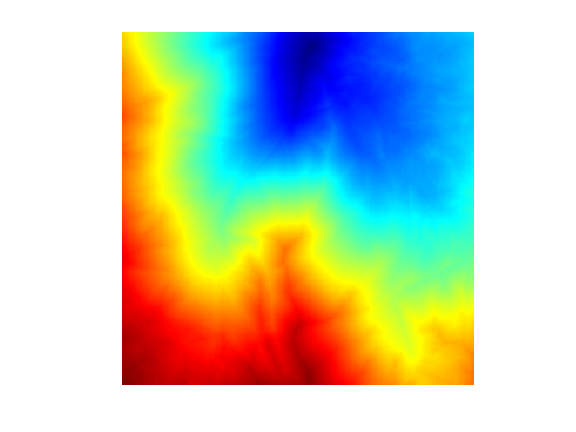Compute the gradient $G_0(x) = \nabla D(x) \in \RR^2$ of the distance map. Use centered differences.

In :
options.order = 2;


Normalize the gradient to obtained $G(x) = G_0(x)/\norm{G_0(x)}$, in order to have unit speed geodesic curve (parameterized by arc length).

In :
G = G0 ./ repmat( sqrt( sum(G0.^2, 3) ), [1 1 2]);


Display $G$.

In :
clf;
imageplot(G);
colormap jet(256);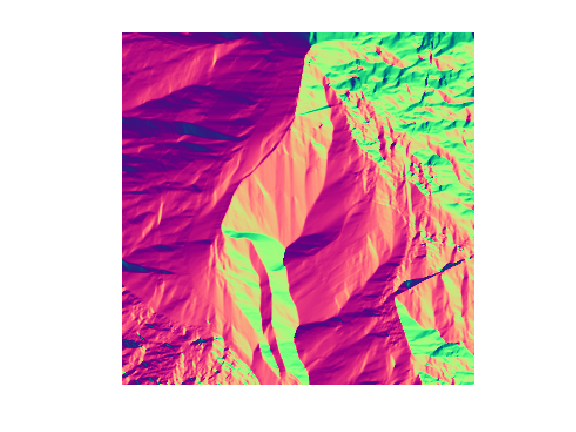The geodesic is then numerically computed using a discretized gradient descent, which defines a discret curve $(\ga_k)_k$ using $$\ga_{k+1} = \ga_k - \tau G(\ga_k)$$ where $\ga_k \in \RR^2$ is an approximation of $\ga(t)$ at time $t=k\tau$, and the step size $\tau>0$ should be small enough.

Step size $\tau$ for the gradient descent.

In :
tau = .8;


Initialize the path with the ending point.

In :
gamma = x1;


Define a shortcut to interpolate $G$ at a 2-D points. Warning: the |interp2| switches the role of the axis ...

In :
Geval = @(G,x)[interp2(1:n,1:n,G(:,:,1),x(2),x(1)); ...
interp2(1:n,1:n,G(:,:,2),x(2),x(1)) ];


Compute the gradient at the last point in the path, using interpolation.

In :
g = Geval(G, gamma(:,end));


Perform the descent and add the new point to the path.

In :
gamma(:,end+1) = gamma(:,end) - tau*g;


Exercise 2

Perform the full geodesic path extraction by iterating the gradient descent. You must be very careful when the path become close to $x_0$, because the distance function is not differentiable at this point. You must stop the iteration when the path is close to $x_0$.

In :
exo2()

In :
%% Insert your code here.


Display the curve on the image background.

In :
clf; hold on;
imageplot(f);
h = plot(gamma(2,:),gamma(1,:), '.b'); set(h, 'LineWidth', 2);
h = plot(x0(2),x0(1), '.r'); set(h, 'MarkerSize', 25);
h = plot(x1(2),x1(1), '.b'); set(h, 'MarkerSize', 25);
axis ij;